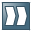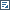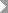Show TOCAdding Values to the Decision Tree Expression

In this step, we will define the different branches of the decision tree object that serves as the tax calculation function's top expression.

Procedure

1. In the Object Manager panel of the application, under the Detail section, choose the Contained Objects tab.

2. In the Contained Objects tab page, choose type Function and selectTAX_CALCULATOR.

The TAX_CALCULATOR function opens in the Object Manager panel.

3. Choose TAX_CALCULATION expression in the Top Expression field.

The decision tree opens in the Object Manager panel.

4. Assign result data object.

1. Next to Result Data Object, choose(Graphical Access).

2. In the context menu, choose Select...

3. In the Object Query dialog box that appears, select the PAYABLE_TAX_AMOUNT data object and choose Select.

The data object is added as a result data object.

5. Assign a root node.

We start the decision tree with a check to find out whether a given income falls into the value range we have defined for low income. To accomplish this, in the context menu of the node, chooseSet Condition...GROSS_SALARY_IS_LOWThe selected expression is set as the root node and two child nodes (positive and negative) appear under the root node as shown below.

6. Add conditions and results to the child node. For the first condition, a positive result indicates that the income in question falls into the range of low income. Because of that, we have to assign the corresponding tax calculation formula for low income to that child node:

1. In the context menu of the positive child node, chooseSet Result...CALCULATE_TAX_FOR_LOW_SALARY.

The expression is added as the result of the child node.

2. In the context menu of the negative child node, we have still to decide if the income in question is medium or high. To model this decision, chooseSet Condition...GROSS_SALARY_IS_MEDIUM.

The expression is added as the condition of the child node.

3. Similarly, add the following expressions as results to the decision tree.

• CALCULATE_TAX_FOR_MED_SALARY

• CALCULATE_TAX_FOR_HIGH_SALARY

7. Save the decision tree.

Next Step

Simulating the Function